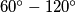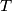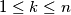### IMO Shortlist 2006 problem C6

Kvaliteta:
Avg: 0,0
Težina:
Avg: 8,0
A holey triangle is an upward equilateral triangle of side length$n$ with$n$ upward unit triangular holes cut out. A diamond is a$60^\circ-120^\circ$ unit rhombus.
Prove that a holey triangle$T$ can be tiled with diamonds if and only if the following condition holds: Every upward equilateral triangle of side length$k$ in$T$ contains at most$k$ holes, for$1\leq k\leq n$.
Izvor: Međunarodna matematička olimpijada, shortlist 2006